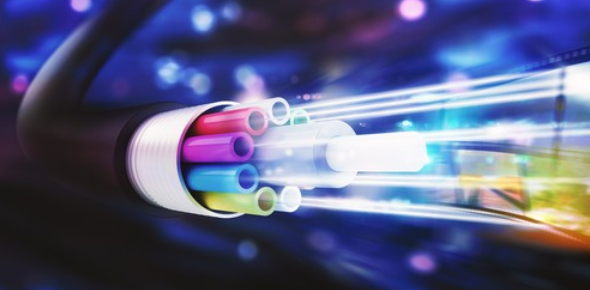# Quiz: Fiber Optics Practice Exam

18 Questions | Attempts: 10030
ShareSettingsHow good you're in fiber optics? Take up this practice exam and see for yourself. Also, this quiz will make you learn about different Fiber optics topics. A fiber optic cable can contain various glasses through which light pulses get through for information to be transferred. There is much that goes into ensuring that the information being spread is transferred at great speeds. Give this quiz a try and get to refresh your memories. Good luck!

• 1.
What does LASER stand for?
• A.

Light Amplification by Simulated Emission of Radiation

• B.

• C.

Laser Amplification by Simulated Emission of Radiation

• D.

None of the above

• 2.
Focal length is determined by?
• A.

The distance from the laser to the lens

• B.

The distance from the battery to the point where the light converges

• C.

The distance from the center of the lens to the point where the light converges

• D.

None of the above

• 3.
Refraction is when?
• A.

Light bends towards the normal

• B.

Light bends away from the normal

• C.

Light becomes one central dot

• D.

Light reflects back from the object

• 4.
Diffraction is when?
• A.

Light bends towards the normal

• B.

Light bends away from the normal

• C.

Light becomes one central dot

• D.

Light reflects back from the object

• 5.
What happens when a laser is pointed into a prism?
• A.

The light shines straight through the prism

• B.

The light counteracts and shines in the opposite direction

• C.

The light shines out the the same side but at a small angle

• D.

All of the above

• 6.
Refraction happens because?
• A.

The light slows down in a material

• B.

The light changes color in a material

• C.

The light speeds up in a material

• D.

The light stops in a material

• 7.
Fiber Optics is
• A.

Communication over copper wires

• B.

Communication over speaker wire

• C.

Communication over thin stands of glass

• D.

All of the above

• 8.
What application does fiber optics not include?
• A.

LANS

• B.

Utilities

• C.

CATV

• D.

Desktop Publishing

• 9.
Why is Fiber Optics used?
• A.

Security

• B.

Speed

• C.

Distance

• D.

Freedom from Interference

• 10.
Outside plant premises is?
• A.

Installing fiber optics in a LAN

• B.

Installing fiber optics outside

• C.

Installing fiber optics in a school

• D.

None of the above

• 11.
The angle of diffraction refers to?
• A.

The change in angle of the diffraction of a laser light

• B.

The change in direction of the laser light

• C.

The change in color of the laser light

• D.

None of the above

• 12.
The core of a fiber optic cable is composed
• A.

Copper wiring

• B.

Thin strands of glass

• C.

Sound waves

• D.

All of the above

• 13.
The light used in Fiber Optic cabling is
• A.

UV

• B.

Visible

• C.

Infrared

• D.

Invisible

• 14.
What is the approx. wavelength of light used in Fiber Optics?
• A.

850 nanometers

• B.

1000 nanometers

• C.

1011 nanometers

• D.

1200 nanometers

• E.

1300 nanometers

• 15.
What is the purpose of the demultiplexer in fiber optics?
• A.

It multiplies the intensity of the light

• B.

It reduces the intensity of the light

• C.

It refracts the light into the correct optical fiber locations

• D.

None of the above

• 16.
What is a fiber optic connector?
• A.

A splice with two wires

• B.

A component created to convert data

• C.

A component designed to protect the glass strands

• D.

None of them

• 17.
Total internal reflection relates to?
• A.

The angle of the light reflecting in the core of the fiber

• B.

The ability to hold in all light sources to speed up the data

• C.

The bouncing back of the light within the fiber core.

• D.

All of the above

• 18.
What is the purpose of the cladding on the fiber cables?
• A.

To prevent light from escaping

• B.

Total internal reflection

• C.

Both a and b

• D.

None of the above

## Related TopicsBack to top
×

Wait!
Here's an interesting quiz for you.